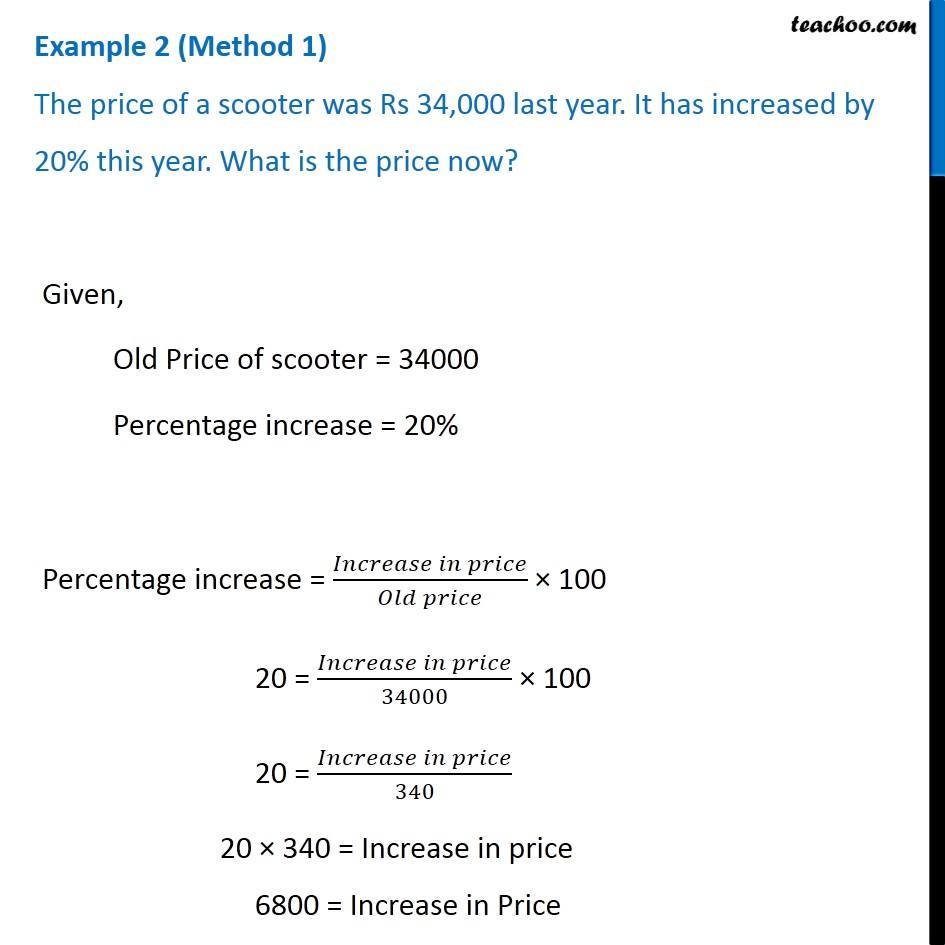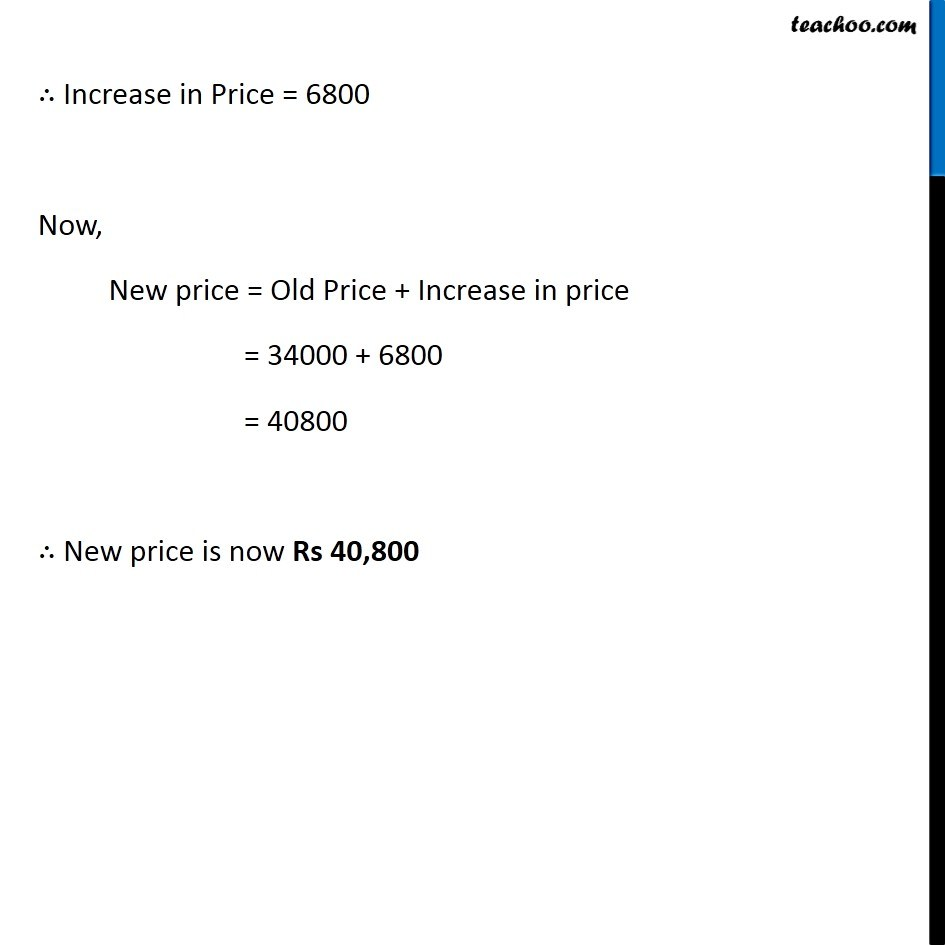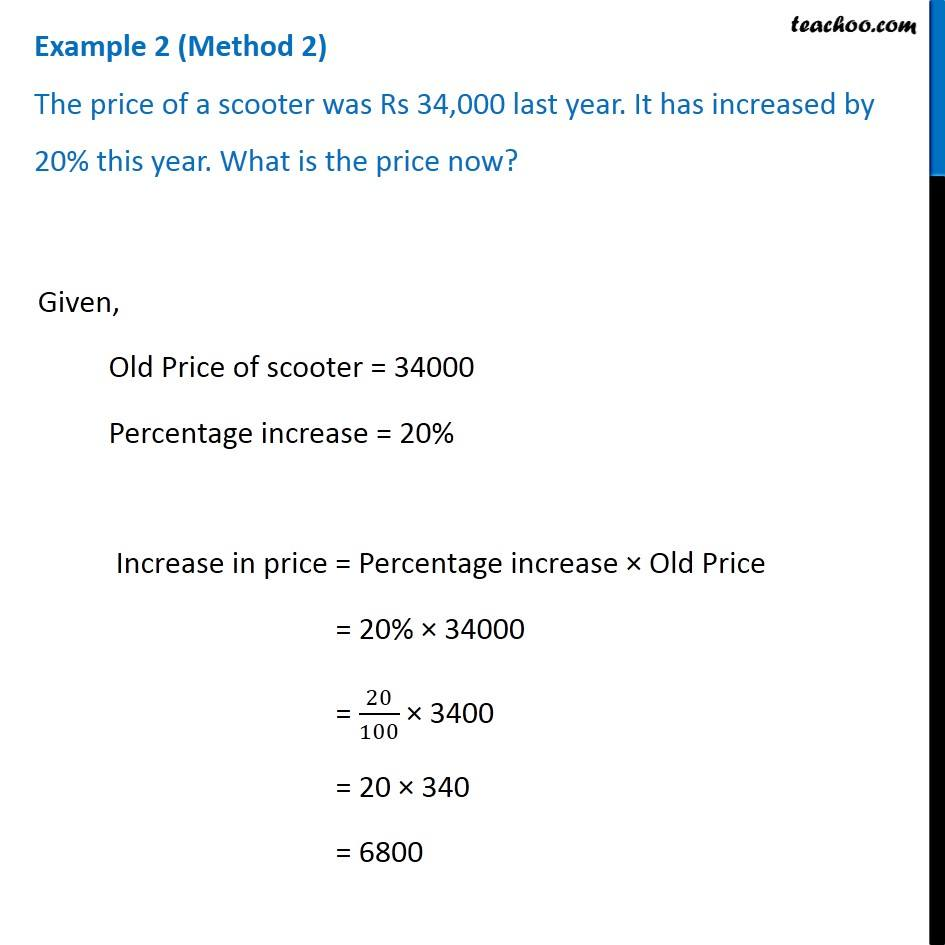Examples

Chapter 7 Class 8 Comparing Quantities
Serial order wiseLearn in your speed, with individual attention - Teachoo Maths 1-on-1 Class

### Transcript

Question 1 (Method 1) The price of a scooter was Rs 34,000 last year. It has increased by 20% this year. What is the price now? Given, Old Price of scooter = 34000 Percentage increase = 20% Percentage increase = (𝐼𝑛𝑐𝑟𝑒𝑎𝑠𝑒 𝑖𝑛 𝑝𝑟𝑖𝑐𝑒)/(𝑂𝑙𝑑 𝑝𝑟𝑖𝑐𝑒) × 100 20 = (𝐼𝑛𝑐𝑟𝑒𝑎𝑠𝑒 𝑖𝑛 𝑝𝑟𝑖𝑐𝑒)/34000 × 100 20 = (𝐼𝑛𝑐𝑟𝑒𝑎𝑠𝑒 𝑖𝑛 𝑝𝑟𝑖𝑐𝑒)/340 20 × 340 = Increase in price 6800 = Increase in Price ∴ Increase in Price = 6800 Now, New price = Old Price + Increase in price = 34000 + 6800 = 40800 ∴ New price is now Rs 40,800 Question 1 (Method 2) The price of a scooter was Rs 34,000 last year. It has increased by 20% this year. What is the price now? Given, Old Price of scooter = 34000 Percentage increase = 20% Increase in price = Percentage increase × Old Price = 20% × 34000 = 20/100 × 3400 = 20 × 340 = 6800 ∴ Increase in Price = 6800 Now, New price = Old Price + Increase in price = 34000 + 6800 = 40800 ∴ New price is now Rs 40,800